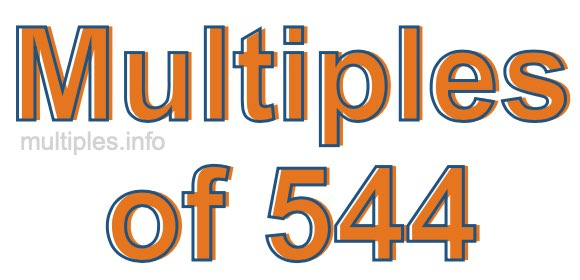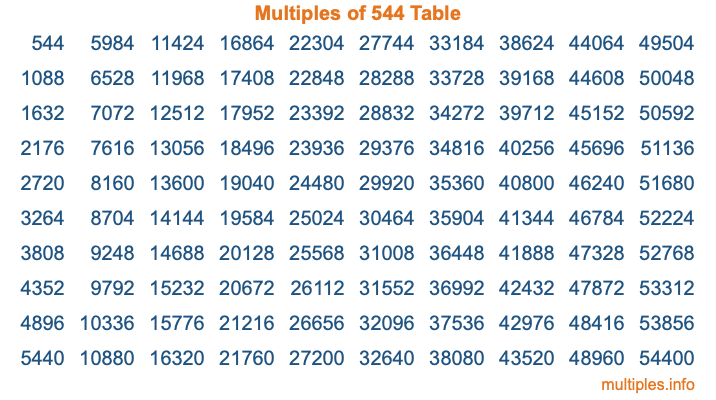Multiples of 544Welcome to the Multiples of 544 page. Here we will first teach you everything you will ever need to know about the multiples of 544, and then give you a study guide summary of everything we taught you to make sure you remember it all. Use this page to look up facts and learn information about the multiples of 544. This page will make you a multiples of five hundred forty-four expert!

Definition of Multiples of 544
Multiples of 544 are all the numbers that when divided by 544 equal an integer. Each of the multiples of 544 are called a multiple. A multiple of 544 is created by multiplying 544 by an integer.

Therefore, to create a list of multiples of 544, you start with 1 multiplied by 544, then 2 multiplied by 544, then 3 multiplied by 544, and so on for as long as you want. Thus, the list of the first five multiples of 544 is 544, 1088, 1632, 2176, and 2720. To see a larger list of multiples of 544, see the printable image of Multiples of 544 further down on this page. We also have a category where you can choose any nth multiple of 544.

Multiples of 544 Checker
The Multiples of 544 Checker below checks to see if any number of your choice is a multiple of 544. In other words, it checks to see if there is any number (integer) that when multiplied by 544 will equal your number. To do that, we divide your number by 544. If the the quotient is an integer, then your number is a multiple of 544.

Is  a multiple of 544?

Least Common Multiple of 544 and ...
A Least Common Multiple (LCM) is the lowest multiple that two or more numbers have in common. This is also called the smallest common multiple or lowest common multiple and is useful to know when you are adding our subtracting fractions. Enter one or more numbers below (544 is already entered) to find the LCM.

Check out our LCM Calculator if you need more details about the Least Common Multiple or if you need the LCM for different numbers for adding and subtraction fractions.

nth Multiple of 544
As we stated above, 544 is the first multiple of 544, 1088 is the second multiple of 544, 1632 is the third multiple of 544, and so on. Enter a number below to find the nth multiple of 544.

th multiple of 544

Multiples of 544 vs Factors of 544
544 is a multiple of 544 and a factor of 544, but that is where the similarities end. All postive multiples of 544 are 544 or greater than 544. All positive factors of 544 are 544 or less than 544.

Below is the beginning list of multiples of 544 and the factors of 544 so you can compare:

Multiples of 544: 544, 1088, 1632, 2176, 2720, etc.

Factors of 544: 1, 2, 4, 8, 16, 17, 32, 34, 68, 136, 272, 544

As you can see, the multiples of 544 are all the numbers that you can divide by 544 to get a whole number. The factors of 544, on the other hand, are all the whole numbers that you can multiply by another whole number to get 544.

It's also interesting to note that if a number (x) is a factor of 544, then 544 will also be a multiple of that number (x).

Multiples of 544 vs Divisors of 544
The divisors of 544 are all the integers that 544 can be divided by evenly. Below is a list of the divisors of 544.

Divisors of 544: 1, 2, 4, 8, 16, 17, 32, 34, 68, 136, 272, 544

The interesting thing to note here is that if you take any multiple of 544 and divide it by a divisor of 544, you will see that the quotient is an integer.

Multiples of 544 Table
Below is an image of the first 100 multiples of 544 in a table. The table is in chronological order, column by column. The first column has the first ten multiples of 544, the second column has the next ten multiples of 544, and so on.The Multiples of 544 Table is also referred to as the 544 Times Table or Times Table of 544. You are welcome to print out our table for your studies.

Negative Multiples of 544
Although not often discussed or needed in math, it is worth mentioning that you can make a list of negative multiples of 544 by multiplying 544 by -1, then by -2, then by -3, and so on, to get the following list of negative multiples of 544:

-544, -1088, -1632, -2176, -2720, etc.

Multiples of 544 Summary
Below is a summary of important Multiples of 544 facts that we have discussed on this page. To retain the knowledge on this page, we recommend that you read through the summary and explain to yourself or a study partner why they hold true.

There are an infinite number of multiples of 544.

A multiple of 544 divided by 544 will equal a whole number.

544 divided by a factor of 544 equals a divisor of 544.

The nth multiple of 544 is n times 544.

The largest factor of 544 is equal to the first positive multiple of 544.

544 is a multiple of every factor of 544.

544 is a multiple of 544.

A multiple of 544 divided by a divisor of 544 equals an integer.

544 divided by a divisor of 544 equals a factor of 544.

Any integer times 544 will equal a multiple of 544.

Multiples of a Number
Here you can get the multiples of another number, all with the same attention to detail as we did for multiples of 544 on this page.

Multiples of
Multiples of 545
Did you find our page about multiples of five hundred forty-four educational? Do you want more knowledge? Check out the multiples of the next number on our list!